# MISSION BANKING 2023 Quantitative Aptitude Quiz- 2

## MISSION BANKING 2023 Quantitative Aptitude Quiz

Quantitative Aptitude is a very essential and scoring section of almost every Banking Exam. To help you all score better in the exams, we are providing you with the MISSION BANKING 2023 Quantitative Aptitude Quiz. In this MISSION BANKING 2023 Quantitative Aptitude Quiz, candidates will be given a full explanation of each question. This MISSION BANKING 2023 Quantitative Aptitude Quiz contains a variety of questions ranging from easy to difficult level. This MISSION BANKING 2023 Quantitative Aptitude Quiz includes all of the most recent pattern-based questions, as well as Previous Year Quantitative Aptitude Questions from banking exams. This MISSION BANKING 2023 Quantitative Aptitude Quiz is available to you at no cost. This MISSION BANKING 2023 Quantitative Aptitude Quiz will assist aspirants in better understanding the topic and learning how to solve tricky problems. Candidates must try to solve this MISSION BANKING 2023 Quantitative Aptitude Quiz to enhance their preparation for upcoming exams.

1. I. 3x² – 28x + 64 = 0

5y² – 33y + 54 = 0

(a) If x<y

(b) If x>y

(c) If x≥y

(d) If x≤y

(e) If x=y or no relation can be established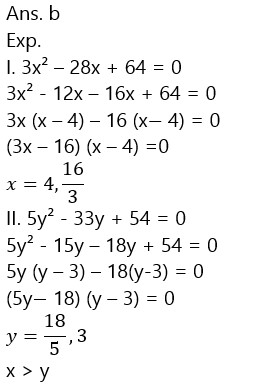1. I. 3x² – 38x + 120 = 0

4y² – 46y + 130 = 0

(a) If x<y

(b) If x>y

(c) If x≥y

(d) If x≤y

(e) If x=y or no relation can be established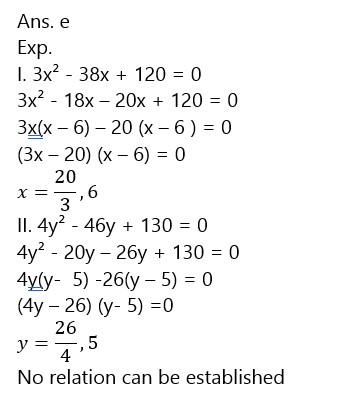1. I. 6x² – 7x – 98 = 0

5y² – 57y + 160 = 0

(a) If x<y

(b) If x>y

(c) If x≥y

(d) If x≤y

(e) If x=y or no relation can be established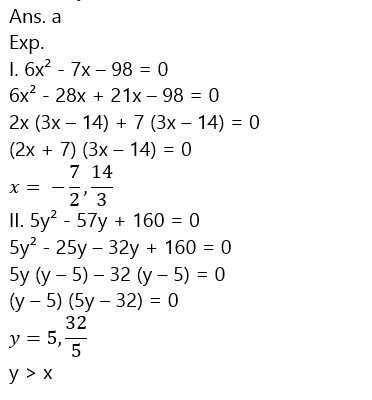1. I. x² – 21x + 108 = 0

y² – 15y + 54 = 0

(a) If x<y

(b) If x>y

(c) If x≥y

(d) If x≤y

(e) If x=y or no relation can be established1. 7x² – 52x + 96 = 0

6y² – 59y + 140 = 0

(a) If x<y

(b) If x>y

(c) If x≥y

(d) If x≤y

(e) If x=y or no relation can be established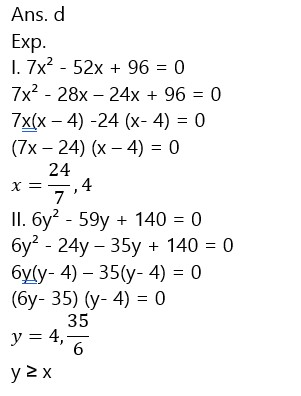1. Sam invested 16000 Rs. in simple interest for 2 years on certain rate and gets an interest of 4800 Rs, if he invested total amount (Principle + Interest) in a scheme, which offered compound interest on 5% more interest rate as earlier rate. Then find total interest gets by Sam after 2 years ?

(a) 9252 Rs.

(b) 9225 Rs.

(c) 9512 Rs.

(d) 925 Rs.

(e) 9152 Rs.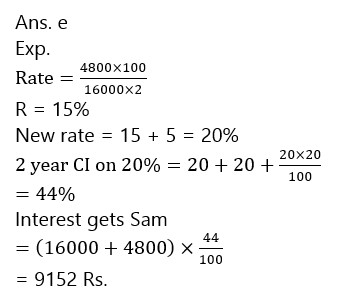1. A can do a task in 48 days B is 25% less efficient than A and C can do the same task in 40 less days as B do. Then find in how many days all three complete task together ?

(a)  64/5  days

(b)  69/5  days

(c)  59/5  days

(d)  79/5  days

(e)  84/5  days1. P can do a piece of work in 72 days and Q can do same work in 48 days, R is 50% more efficient than Q then find in how many days total work will be complete if all three work alternatively days starting with P and ending with R ?

(a)  175/4 days

(b)  183/4 days

(c)  187/4 days

(d)  171/4 days

(e)  191/4 days1. A shopkeeper sold an article at a 10% discount at mark price. He found that he earns a profit of 50/3% but instead of calculating profit on cost price he calculates it on the sum of cost price and selling price. If cost price of article is 1350 then find out the mark price.

(a) 2100

(b) 2300

(c) 1890

(d) 1500

(e) None of these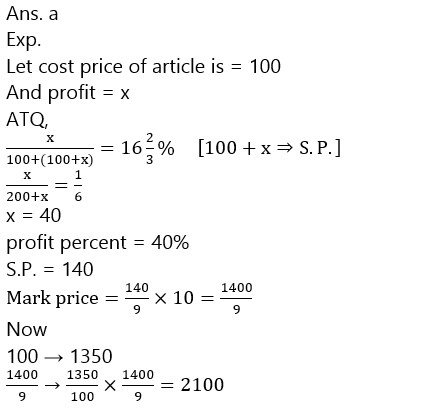1. Two types of alloy possess gold and silver in the ratio of 7 : 22 and 21 : 37. In what ratio should these alloys be mixed so as to have a new alloy in which gold and silver would exist in the ratio 25 : 62?

(a) 13 : 8

(b) 8 : 13

(c) 13 : 12

(d) 6 : 9

(e) None of these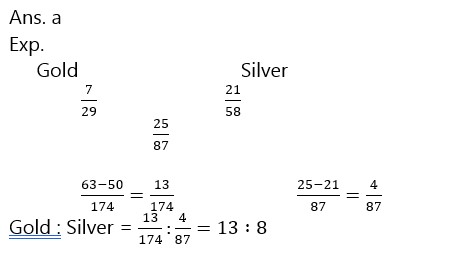### 2023 Preparation Kit PDF

#### Most important PDF’s for Bank, SSC, Railway and Other Government Exam : Download PDF Now

AATMA-NIRBHAR Series- Static GK/Awareness Practice Ebook PDF Get PDF here
The Banking Awareness 500 MCQs E-book| Bilingual (Hindi + English) Get PDF here
AATMA-NIRBHAR Series- Banking Awareness Practice Ebook PDF Get PDF here
Computer Awareness Capsule 2.O Get PDF here
AATMA-NIRBHAR Series Quantitative Aptitude Topic-Wise PDF 2020 Get PDF here
Memory Based Puzzle E-book | 2016-19 Exams Covered Get PDF here
Caselet Data Interpretation 200 Questions Get PDF here
Puzzle & Seating Arrangement E-Book for BANK PO MAINS (Vol-1) Get PDF here
ARITHMETIC DATA INTERPRETATION 2.O E-book Get PDF here

3